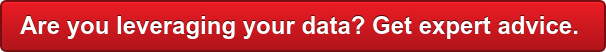# What are “Finite Mixture Models?”​

Many marketing researchers and data scientists may have heard of finite mixture models (FMM) but are not clear what they are. This is understandable since articles and online discussions about technical subjects such as FMM often confuse more than enlighten.

It’s also unfortunate because FMM are useful tools for researchers and data analysts in many fields. They are complex, but no longer obscure, and have real practical value – I have used them for many years.

So, what are FMM? Here is a concise definition adapted from the Stata 16 software manual:

“A finite mixture model (FMM) is a statistical model that assumes the presence of unobserved groups, called latent classes, within an overall population. Each latent class can be fit with its own regression model, which may have a linear or generalized linear response function.

We can compare models with differing numbers of latent classes and different sets of constraints on parameters to determine the best fitting model.

For a given model, we can compare parameter estimates across classes. We can estimate the proportion of the population in each latent class, and we can predict the probabilities that the observations in our sample belong to each latent class.”

A common mistake in statistical modelling is to assume “one size fits all” or to run multiple models on subjectively pre-defined subgroups of consumers, for example. FMM often reveal unexplained heterogeneity that is either ignored or only partially revealed by multiple models for a priori groups.

I should note that there are also advanced variations of FMM which are extensions of factor analysis and structural equation modelling (SEM).

For those seeking a bit more technical detail regarding FMM, I’ve included some entries from the manual’s glossary on FMM below.

Any copy/paste and editing errors are mine.

___________________________________________________________categorical latent variable. A categorical latent variable has levels that represent unobserved groups in the population. Latent classes are identified with the levels of the categorical latent variables and may represent healthy and unhealthy individuals, consumers with different buying preferences, or different motivations for delinquent behaviour.

class model. A class model is a regression model that is applied to one component in a mixture model. In the absence of covariates, the regression model reduces to a distribution function. Class model is also referred to in the literature as a “component model”, “component density”, or “component distribution”.

class probability. In the context of FMM, the probability of belonging to a given class. FMM uses multinomial logistic regression to model class probabilities. Class probability is also referred to in the literature as a “latent class probability”, “component probability”, “mixture component probability”, “mixing probability”, “mixing proportion”, “mixing weight”, or “mixture probability”.

expectation-maximization algorithm. In the context of FMM, an iterative procedure for refining starting values before maximizing the likelihood. The EM algorithm uses the complete-data likelihood as if we have observed values for the latent class indicator variable.

generalized linear response functions. Generalized linear response functions include linear functions and include functions such as probit, logit, multinomial logit, ordered probit, ordered logit, Poisson, and more. In this generalized linear structure, the family may be Gaussian, gamma, Bernoulli, binomial, Poisson, negative binomial, ordinal, or multinomial. The link function may be the identity, log, logit, probit, or complementary log-log.

latent class. A latent class is an unobserved group identified by a level of a categorical latent variable. Latent class is also referred to in the literature as a “class”, “group”, “type”, or “mixture component”.

pointmass density. In the context of FMM, a degenerate distribution that takes on a single integer value with probability one. A pointmass density is used in combination with other FMM distributions to model, most commonly, zero-inflated outcomes.

Those with some background in programming may also find these Stata examples helpful:

Mixture of three normal distributions of y:

fmm 3: regress y

Mixture of three linear regression models of y on x1 and x2:

fmm 3: regress y x1 x2

As above, but with class probabilities depending on z1 and z2:

fmm 3, lcprob(z1 z2): regress y x1 x2

As above, but with additional class-specific regression covariates x3, x4, and x5:

fmm, lcprob(z1 z2): (regress y x1 x2 x3)

(regress y x1 x2 x4)

(regress y x1 x2 x5);

As above, but with additional class-specific probability covariates z3 and z4:

fmm: (regress y x1 x2 x3)

(regress y x1 x2 x4, lcprob(z1 z2 z3))

(regress y x1 x2 x5, lcprob(z1 z2 z4));

Source: Stata 16 manual, StataCorp

Browse

Article by channel:

Read more articles tagged: Analytics, Featured, Marketing Analytics